Get inspired by the success stories of our students in IIT JAM MS, ISI  MStat, CMI MSc DS.  Learn More

# ISI MStat PSA 2019 Problem 18 | Probability and Digits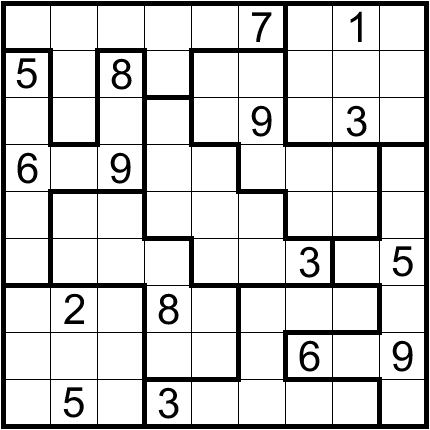This problem is a very easy and cute problem of probability from ISI MStat PSA 2019 Problem 18.

## Probability and Digits - ISI MStat Year 2019 PSA Problem 18

Draw one observationat random from the set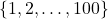. What is the probability that the last digit ofis?

•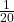•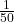•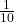•### Prerequisites

Last Digit of Natural Numbers

Basic Probability Theory

Combinatorics

Answer: isISI MStat 2019 PSA Problem Number 18

A First Course in Probability by Sheldon Ross

## Try with Hints

Try to formulate the sample space. Observe that the sample space is not dependent on the number itself rather only on the last digits of the number.

Also, observe that the number of integers inis uniformly distributed over the last digits. So the sample space can be taken as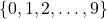. So, the number of elements in the sample space is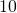.

See the Food for Thought!

This step is easy.

Find out the cases for which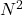gives 1 as the last digit. Use the reduced last digit sample space.

• 1 x 1
• 3 x 7 (Sinceand they must have the same last digit)
• 7 x 3 (Sinceand they must have the same last digit)
• 9 x 9

So, there are 2 possible cases out of 10.

Therefore the probability =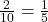.

• Observe that there is a little bit of handwaving in the First Step. Please make it more precise using the ideas of Probability that it is okay to use the sample space as the reduced version rather than.
• Generalize the problem for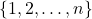.
• Generalize the problems forfor selecting an observation from.
• Generalize the problems forfor selecting an observation fromfor each of the digits from.

## Subscribe to Cheenta at Youtube

This problem is a very easy and cute problem of probability from ISI MStat PSA 2019 Problem 18.

## Probability and Digits - ISI MStat Year 2019 PSA Problem 18

Draw one observationat random from the set. What is the probability that the last digit ofis?

••••### Prerequisites

Last Digit of Natural Numbers

Basic Probability Theory

Combinatorics

Answer: isISI MStat 2019 PSA Problem Number 18

A First Course in Probability by Sheldon Ross

## Try with Hints

Try to formulate the sample space. Observe that the sample space is not dependent on the number itself rather only on the last digits of the number.

Also, observe that the number of integers inis uniformly distributed over the last digits. So the sample space can be taken as. So, the number of elements in the sample space is.

See the Food for Thought!

This step is easy.

Find out the cases for whichgives 1 as the last digit. Use the reduced last digit sample space.

• 1 x 1
• 3 x 7 (Sinceand they must have the same last digit)
• 7 x 3 (Sinceand they must have the same last digit)
• 9 x 9

So, there are 2 possible cases out of 10.

Therefore the probability =.

• Observe that there is a little bit of handwaving in the First Step. Please make it more precise using the ideas of Probability that it is okay to use the sample space as the reduced version rather than.
• Generalize the problem for.
• Generalize the problems forfor selecting an observation from.
• Generalize the problems forfor selecting an observation fromfor each of the digits from.

## Subscribe to Cheenta at Youtube

This site uses Akismet to reduce spam. Learn how your comment data is processed.

### Knowledge Partner